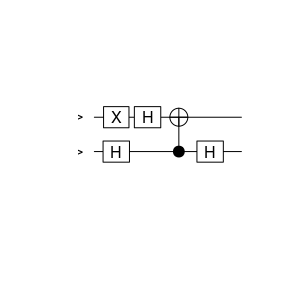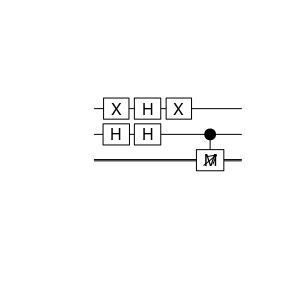# The Deutsch-Jozsa Algorithm

This is an example implementation of the Deutsch-Jozsa algorithm for the special case of $$2$$ qubits. The algorithm allows to distinguish between a constant or balanced function $$f$$ with a single application of $$f$$, relying on what is called quantum parallelism.

We first prepare a state $$|\psi_0\rangle = |x, y\rangle = |0, 1\rangle$$ with only 2 qubits as follows

x <- X(1) * qstate(nbits=2, basis=genComputationalBasis(2, collapse=","))
x
##    ( 1 ) * |0,1>

Note that we count the qubits from one to number of qubits, and the least significant bit (the right most one) is counted first.

Using the Hadamard gate on both qubits results in a superposition in both qubits

y <- H(2) * (H(1) * x)
y
##    ( 0.5 )   * |0,0>
##  + ( -0.5 )  * |0,1>
##  + ( 0.5 )   * |1,0>
##  + ( -0.5 )  * |1,1>

The next step is to apply the uniform transformation $$U_f$$ to the state $$|x\rangle(|0\rangle - |1\rangle)$$. The action of $$U_f$$ was defined as $$|x,y\rangle \to |x, y\oplus f(x)\rangle$$, where $$\oplus$$ is addition modulo $$2$$. The function $$f$$ is a function $$\{0,1\}\to\{0,1\}$$.

We first consider a so-called balanced function $$f(x)$$, i.e. it is equal to $$1$$ for exactly half of the possible $$x$$. In our case with a single qubit $$x$$ this could be $$f(0)=0$$ and $$f(1) = 1$$.

$$U_f$$ is realised in this case by CNOT$$(2,1)$$, where we consider the second qubit as the control qubit. For $$|x, y\oplus f(x)\rangle$$, there are four different possibilities

• $$x=0, y=0$$, $$U_f(|0,0\rangle) = |0, 0\oplus f(0)\rangle = |0, 0\rangle$$
• $$x=1, y=0$$, $$U_f(|1,0\rangle) = |1, 0\oplus f(1)\rangle = |1, 1\rangle$$
• $$x=0, y=1$$, $$U_f(|0,1\rangle) = |0, 1\oplus f(0)\rangle = |0, 1\rangle$$
• $$x=1, y=1$$, $$U_f(|1,1\rangle) = |1, 1\oplus f(1)\rangle = |1, 0\rangle$$

Now,

• CNOT$$(2,1)|0,0\rangle = |0,0\rangle$$
• CNOT$$(2,1)|1,0\rangle = |1,1\rangle$$
• CNOT$$(2,1)|0,1\rangle = |0,1\rangle$$
• CNOT$$(2,1)|1,1\rangle = |1,0\rangle$$

which is what we wanted to archive. Thus, we apply it:

z <- CNOT(c(2, 1)) * y
z
##    ( 0.5 )   * |0,0>
##  + ( -0.5 )  * |0,1>
##  + ( -0.5 )  * |1,0>
##  + ( 0.5 )   * |1,1>

Now apply the Hadamard gate again on $$x$$ (the query register), i.e. the second qubit

u <- H(2) * z
u
##    ( 0.7071068 ) * |1,0>
##  + ( -0.7071068 )    * |1,1>

Now qubit $$2$$ equals $$1$$, thus, if we measure,

value <- measure(u, 2)$value we obtain $$1$$. We can also plot the corresponding circuit plot(u, qubitnames=c("|y>", "|x>"), cbitnames="c")On the other hand, a constant function $$f(x) = 1$$ leads to • $$x=0, y=0$$, $$U_f(|0,0\rangle) = |0, 0\oplus f(0)\rangle = |0, 1\rangle$$ • $$x=1, y=0$$, $$U_f(|1,0\rangle) = |1, 0\oplus f(1)\rangle = |1, 1\rangle$$ • $$x=0, y=1$$, $$U_f(|0,1\rangle) = |0, 1\oplus f(0)\rangle = |0, 0\rangle$$ • $$x=1, y=1$$, $$U_f(|1,1\rangle) = |1, 1\oplus f(1)\rangle = |1, 0\rangle$$ which can be realised with a NOT operation on the first qubit • X$$(1)|0,0\rangle = |0,1\rangle$$ • X$$(1)|1,0\rangle = |1,1\rangle$$ • X$$(1)|0,1\rangle = |0,0\rangle$$ • X$$(1)|1,1\rangle = |1,0\rangle$$ So, the same algorithm again, now with the constant $$f$$ x <- X(1) * qstate(nbits=2, basis=genComputationalBasis(2, collapse=",")) y <- H(2) * (H(1) * x) z <- X(1) * y z ## ( -0.5 ) * |0,0> ## + ( 0.5 ) * |0,1> ## + ( -0.5 ) * |1,0> ## + ( 0.5 ) * |1,1> u <- H(2) * z u ## ( -0.7071068 ) * |0,0> ## + ( 0.7071068 ) * |0,1> v <- measure(u, 2) v ##$bit
##  2
##
## $repetitions ##  1 ## ##$prob
##  0.5 0.5 0.0 0.0
##
## $value ##  0 ## ##$psi
##    ( -0.7071068 )    * |0,0>
##  + ( 0.7071068 ) * |0,1>
##
## attr(,"class")
##  "measurement" "list"
plot(v$psi)value <- v$value

and we obtain $$0$$ for the second qubit.

## Export to Qiskit

In principle the code in Qiskit could look somehow like this:

filename <- paste0(tempdir(), "/circuit.py")
export2qiskit(u, filename=filename)
cat(readLines(filename), sep = '\n')
# automatically generated by qsimulatR
qc = QuantumCircuit(2)
qc.x(0)
qc.h(0)
qc.h(1)
qc.x(0)
qc.h(1)

But this does not include the measurement and the measurement cannot simply be added as an additional command. If a measurement is to be performed, one has to add corresponding classical bits. To do so export the state including the measurement:

export2qiskit(v\$psi, filename=filename, import=TRUE)
cat(readLines(filename), sep = '\n')
# automatically generated by qsimulatR
import numpy as np
from qiskit import(QuantumCircuit, execute, Aer)
from qiskit.visualization import plot_histogram
simulator = Aer.get_backend('qasm_simulator')
qc = QuantumCircuit(2,1)
qc.x(0)
qc.h(0)
qc.h(1)
qc.x(0)
qc.h(1)
qc.measure(1, 0)

Then the results from the Quiskit simulation can be obtained with

qc.draw()

res = execute(qc, simulator, shots=1)
res.result().get_counts(qc)